# Midterm Review for Mathematics

Created
Best for live in-class or video conferencing lessonsStart teacher-led lesson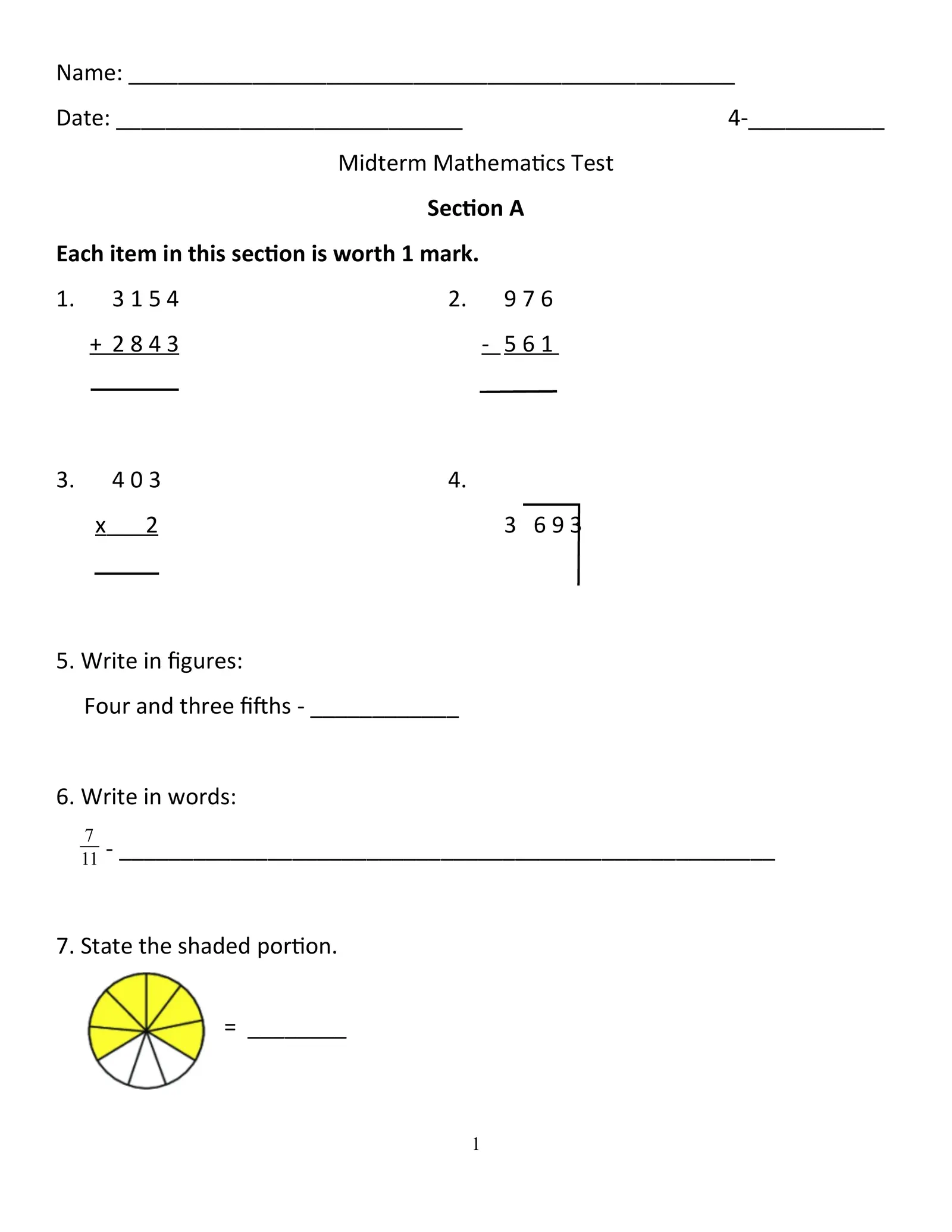Name: _________________________________________________ Date: ____________________________ 4-___________ Midterm Mathematics Test Section A Each item in this section is worth 1 mark. 1. 3154 2. 976 + 2843 - 561 3. 403 4. x 2 3 693 5. Write in figures: Four and three fifths - ____________ 6. Write in words: 7 11 - _____________________________________________________ 7. State the shaded portion. = ________ 1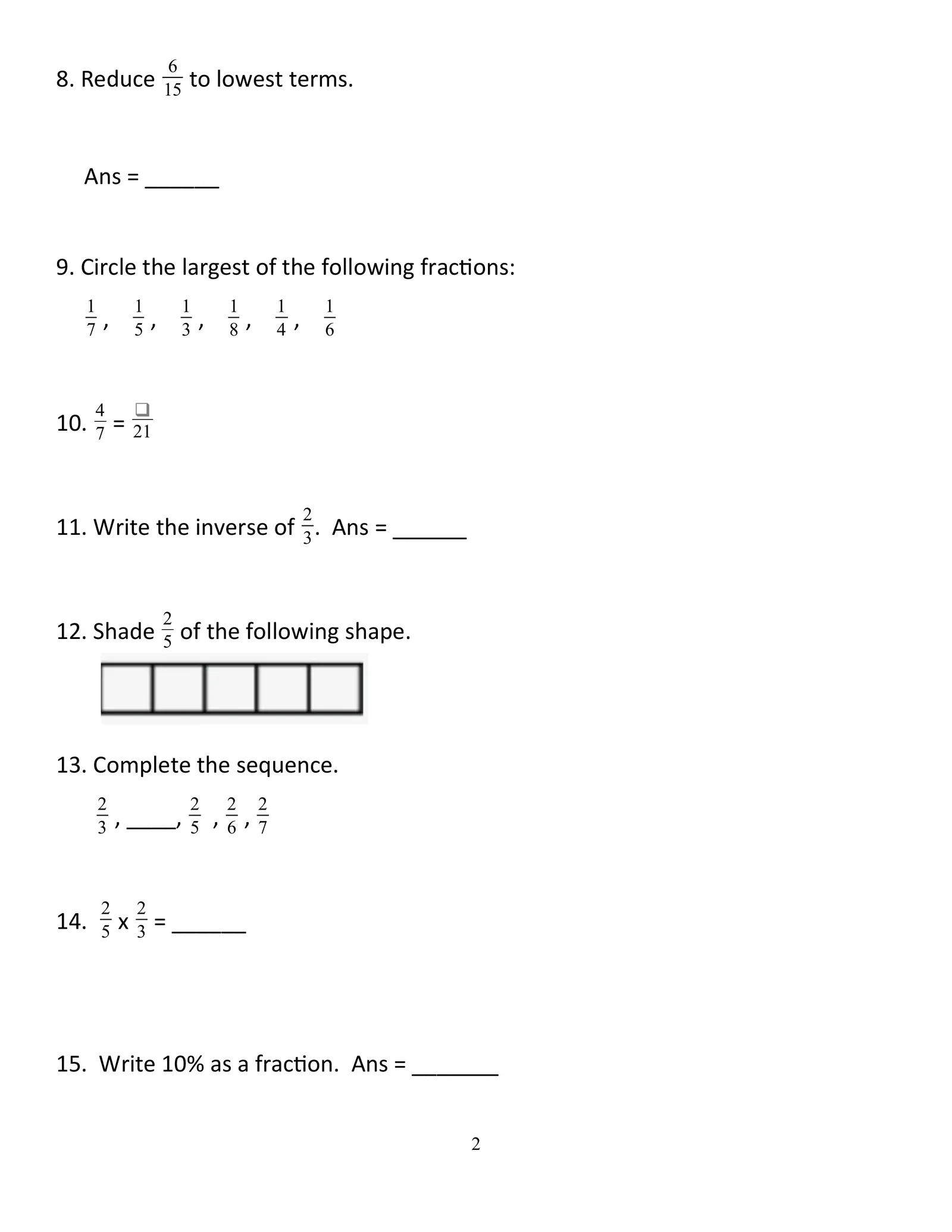6 8. Reduce 15 to lowest terms. Ans = ______ 9. Circle the largest of the following fractions: 1 1 1 1 1 1 7 , 5 , 3 , 8 , 4 , 6 4 ❑ 10. 7 = 21 2 11. Write the inverse of 3 . Ans = ______ 2 12. Shade 5 of the following shape. 13. Complete the sequence. 2 2 2 2 3 , ____, 5 , 6 , 7 2 2 14. 5 x 3 = ______ 15. Write 10% as a fraction. Ans = _______ 2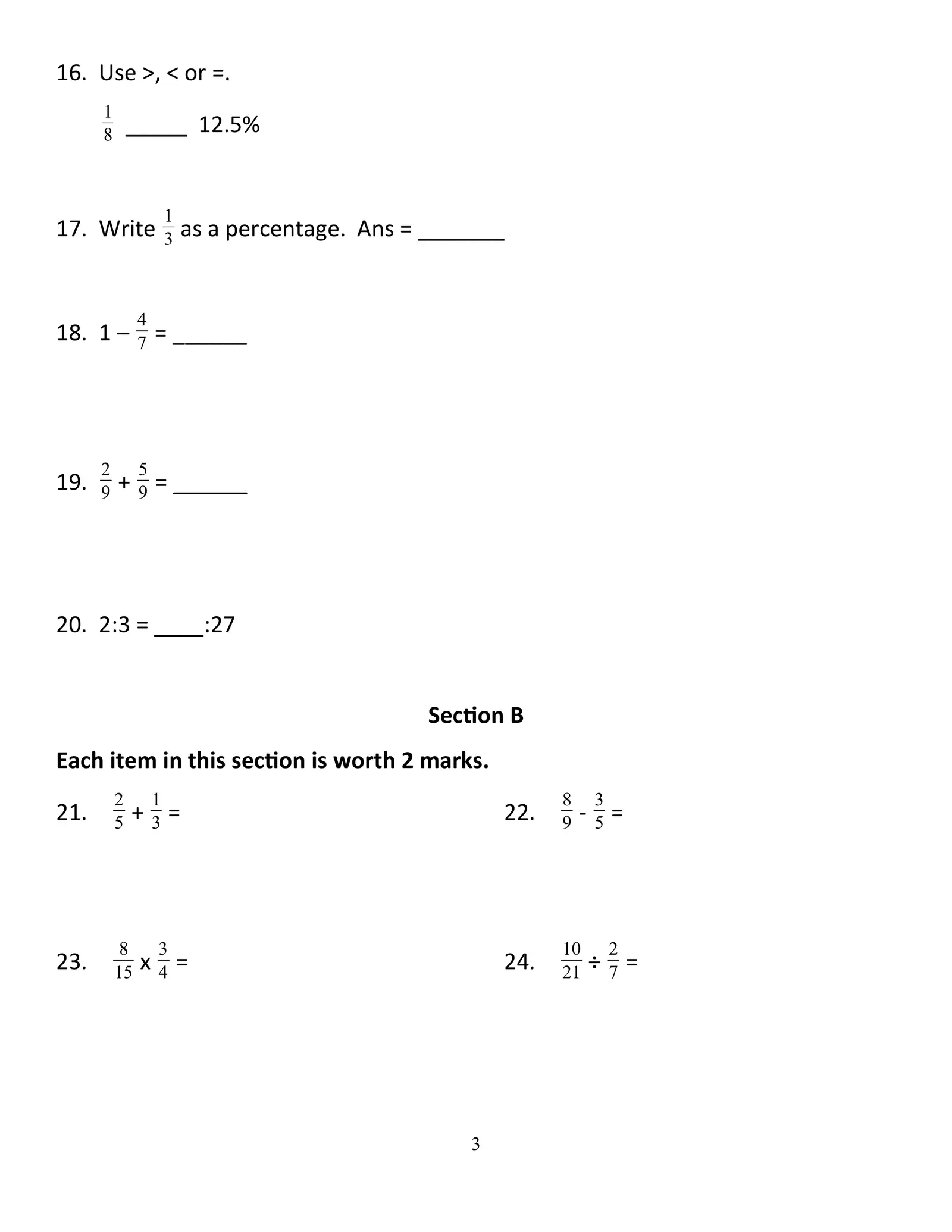16. Use >, < or =. 1 8 _____ 12.5% 1 17. Write 3 as a percentage. Ans = _______ 4 18. 1 – 7 = ______ 2 5 19. 9 + 9 = ______ 20. 2:3 = ____:27 Section B Each item in this section is worth 2 marks. 2 1 8 3 21. 5 +3= 22. 9 -5= 8 3 10 2 23. 15 x 4 = 24. 21 ÷ 7 = 3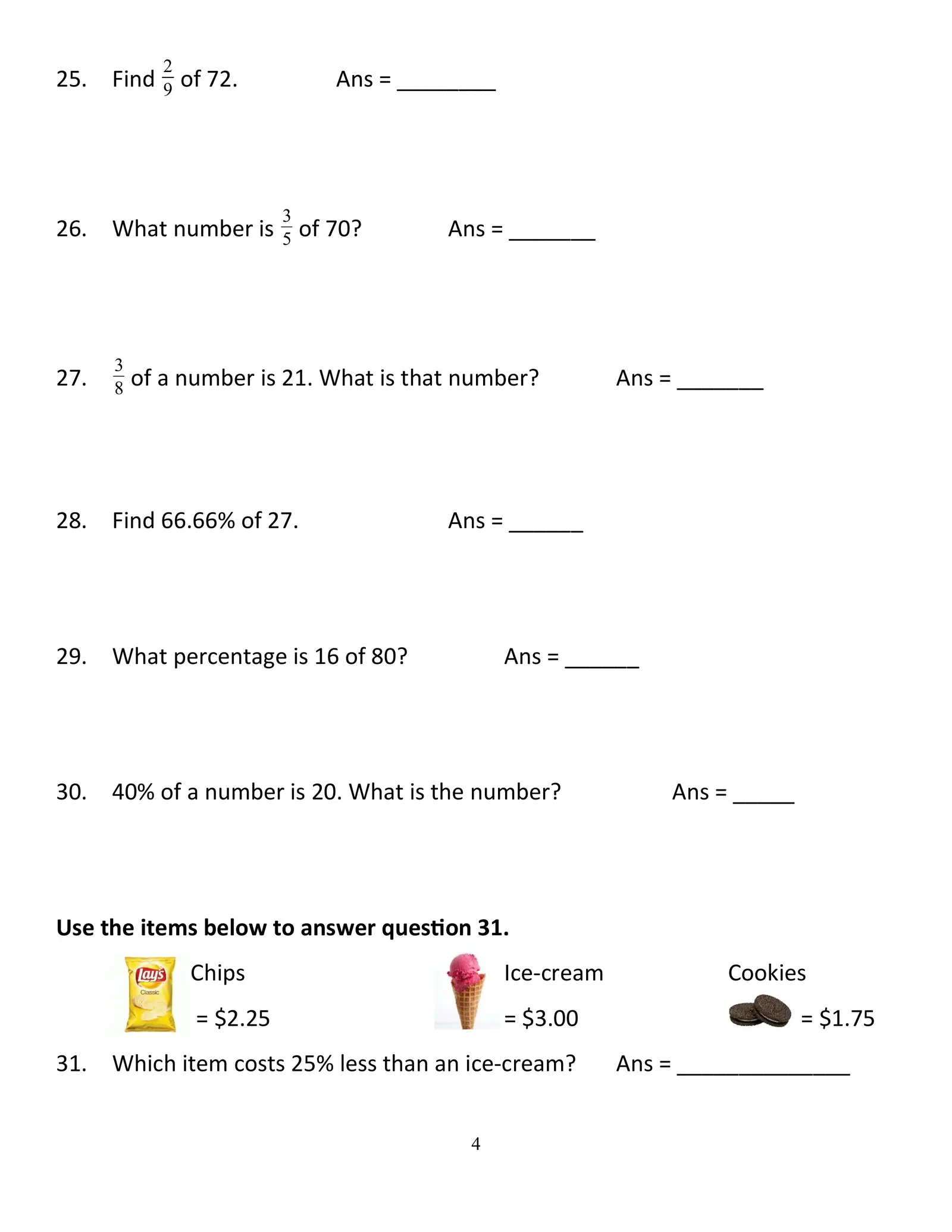2 25. Find 9 of 72. Ans = ________ 3 26. What number is 5 of 70? Ans = _______ 3 27. 8 of a number is 21. What is that number? Ans = _______ 28. Find 66.66% of 27. Ans = ______ 29. What percentage is 16 of 80? Ans = ______ 30. 40% of a number is 20. What is the number? Ans = _____ Use the items below to answer question 31. Chips Ice-cream Cookies = \$2.25 = \$3.00 = \$1.75 31. Which item costs 25% less than an ice-cream? Ans = ______________ 4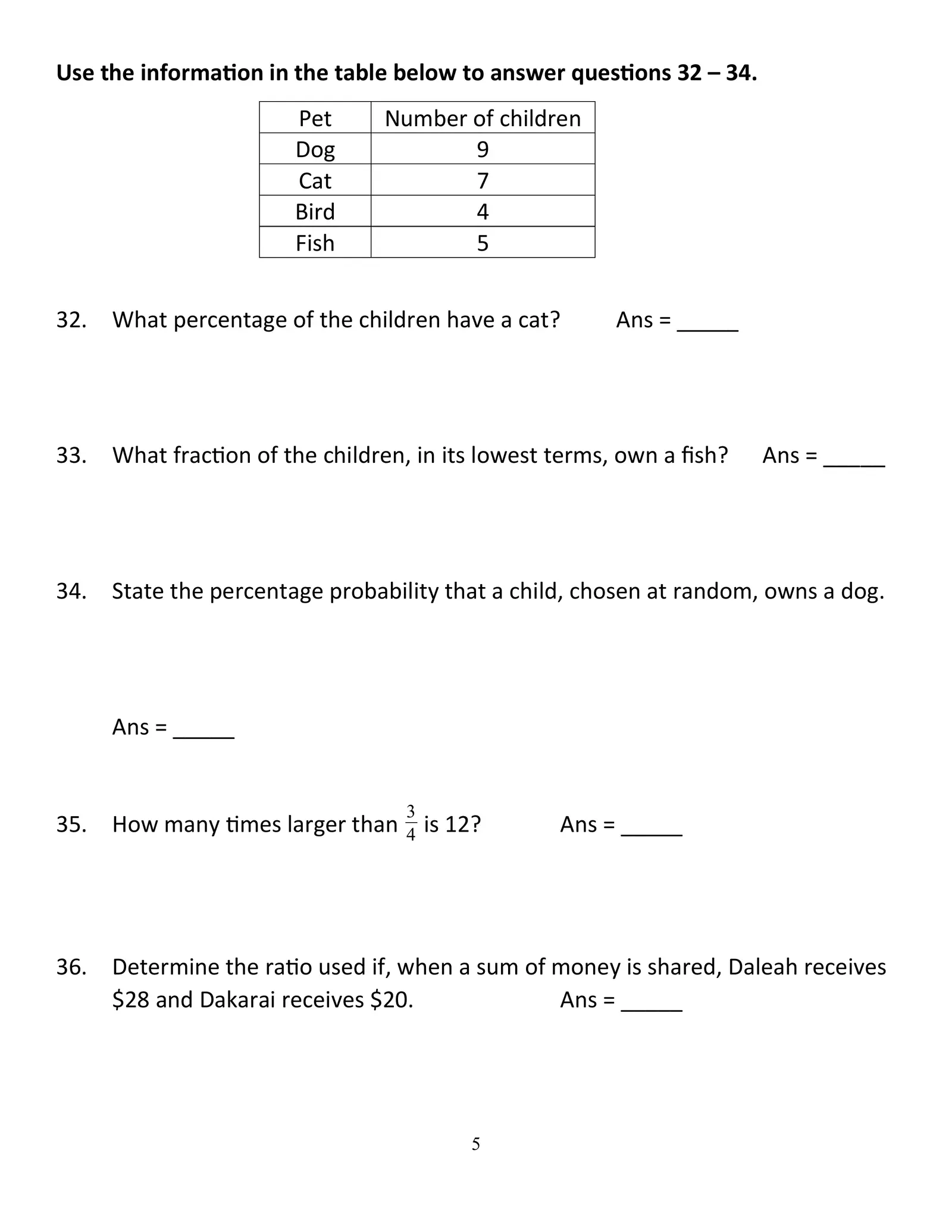Use the information in the table below to answer questions 32 – 34. Pet Number of children Dog 9 Cat 7 Bird 4 Fish 5 32. What percentage of the children have a cat? Ans = _____ 33. What fraction of the children, in its lowest terms, own a fish? Ans = _____ 34. State the percentage probability that a child, chosen at random, owns a dog. Ans = _____ 3 35. How many times larger than 4 is 12? Ans = _____ 36. Determine the ratio used if, when a sum of money is shared, Daleah receives \$28 and Dakarai receives \$20. Ans = _____ 5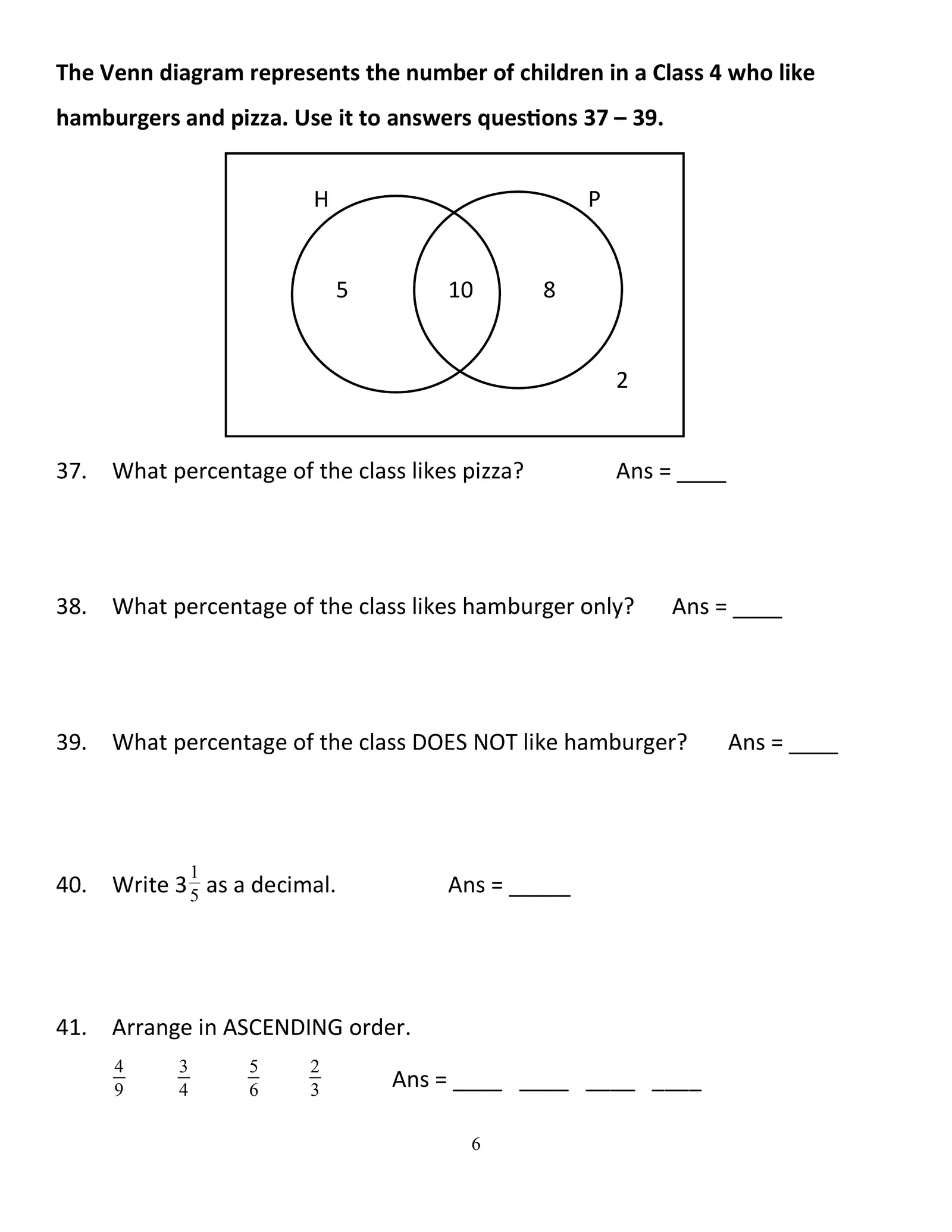The Venn diagram represents the number of children in a Class 4 who like hamburgers and pizza. Use it to answers questions 37 – 39. H P 5 10 8 2 37. What percentage of the class likes pizza? Ans = ____ 38. What percentage of the class likes hamburger only? Ans = ____ 39. What percentage of the class DOES NOT like hamburger? Ans = ____ 1 40. Write 3 5 as a decimal. Ans = _____ 41. Arrange in ASCENDING order. 4 3 5 2 9 4 6 3 Ans = ____ ____ ____ ____ 6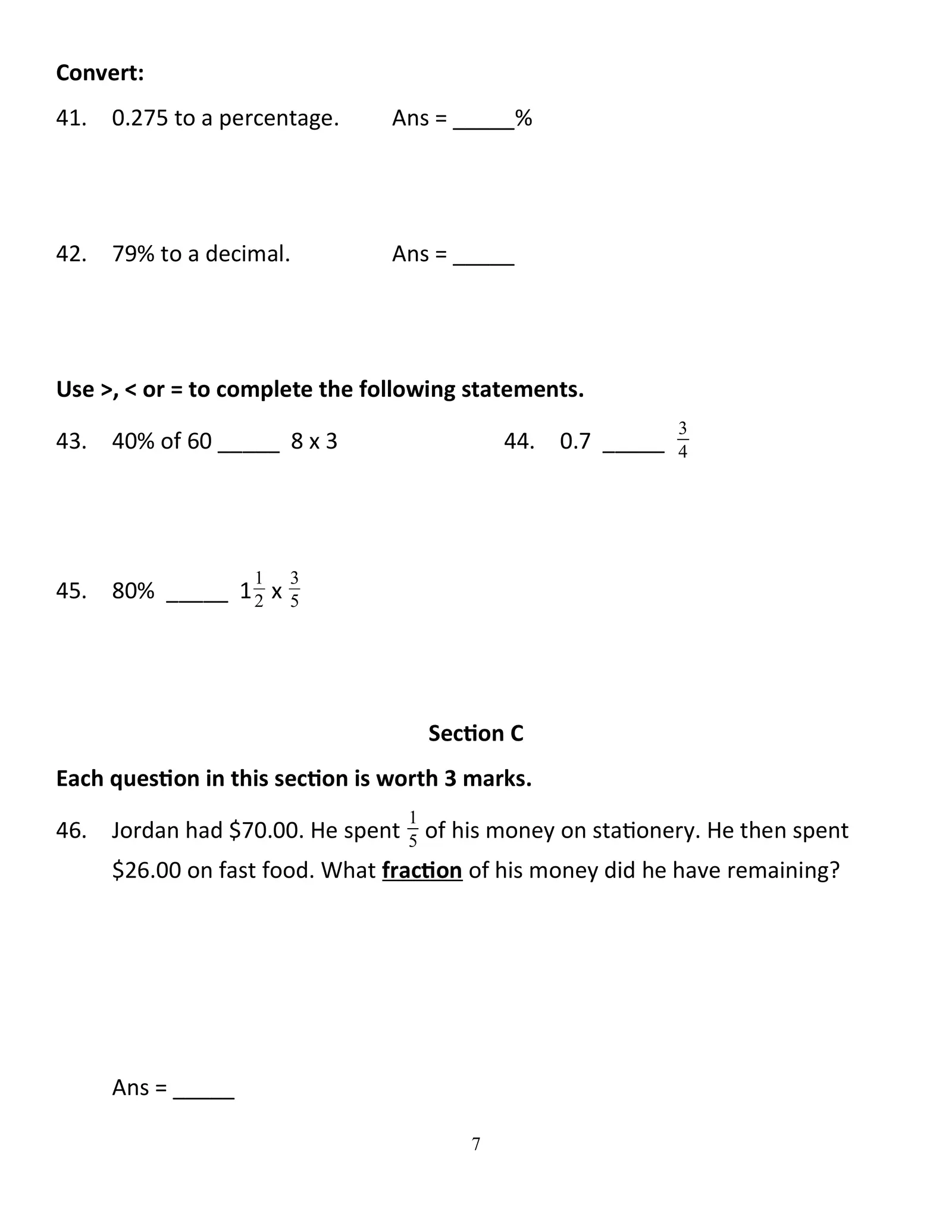Convert: 41. 0.275 to a percentage. Ans = _____% 42. 79% to a decimal. Ans = _____ Use >, < or = to complete the following statements. 3 43. 40% of 60 _____ 8 x 3 44. 0.7 _____ 4 1 3 45. 80% _____ 1 2 x 5 Section C Each question in this section is worth 3 marks. 1 46. Jordan had \$70.00. He spent 5 of his money on stationery. He then spent \$26.00 on fast food. What fraction of his money did he have remaining? Ans = _____ 7